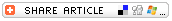Home >> A Tsl >> Archives >> 02 1 >> Counting with Marshmallows and Licorice

Search form

Home > Teacher Lesson Plans > Archives > Lesson Plan

L E S S O N     P L A N

Counting With Marshmallows and LicoriceSubjects:

Algebra, Arithmetic

Pre-K, K-2

Note

Before preparing or distributing any food in the classroom, make sure you're aware of children's allergies or dietary restrictions and caution children about choking hazards.

Brief Description

This activity helps students count using tens and ones in a "yummy" way. The activity should work well with young students and in special education classes.

Objectives

Students

• use snack foods to count and add into the double digits.
• use plastic tens and ones blocks to count and add in the double digits.

Keywords

addition, education, math, nutrition, ones, place value, snack, special, tens

Materials Needed

• licorice sticks
• mini-marshmallows
• paper
• pencil
• plastic tens and ones blocks

Lesson Plan

Start the lesson by reviewing counting skills and basic addition to 10. Then provide each student with ten mini-marshmallows. Use the marshmallows for counting exercises: for example, students count out the number of marshmallows that equals their ages, their grade, or the number of siblings they have. Then introduce simple addition problems. Students use the marshmallows to perform simple addition up to a sum of 10: for example, 2 marshmallows+ 5 marshmallows = __ marshmallows.

Next, have students make a "math mat." The math mat is simply a piece of paper with nine squares or rectangles. Students will be able to use this math mat to add two two-digit numbers and, eventually, three-digit numbers that add up to 999 or less. The mat's columns will look like this (each X represents a square):

 XXX
 +XXX
_____
 =XXX

Teach students to use the math mat to solve simple addition problems that add to a sum of 10 or less (for example, 4 + 4 = __ marshmallows).

Now introduce a licorice stick for counting numbers or sums greater than 10. Introduce the idea that a licorice stick is the same value as ten marshmallows; ten marshmallows could be "traded" for one licorice stick. Practice counting and showing a licorice-marshmallow representation for numbers greater than 10. For example, a licorice stick and four marshmallows represents the number 14.

Next, challenge students to use their math mats to solve a simple addition problem that results in a sum of 10 or greater: for example, 6 marshmallows + 5 marshmallows = __ marshmallows. They will quickly discover they do not have enough marshmallows to work the problem. But they can "trade" ten marshmallows for a licorice stick. The licorice sticks will always appear in the second column (the tens column) on their math charts. Practice adding numbers that result in a sum greater than 10 but less than 99.

Eventually, as students learn to add to numbers greater than 99, introduce a pretzel or a carrot stick to represent ten licorice sticks.

End the lesson by enjoying a tasty, low-fat snack.

Assessment

Use tens and ones plastic math blocks to check transferability of students' place value skills from food to real math manipulatives.

Submitted By

Lisa Miller, Riverside Elementary School, Toledo, Ohio

Originally published 01/18/2002
Updated 04/14/2008To help us keep our Lesson Plan Database as current as possible, please e-mail us to report any links that are not working.